# ILP Part 52 — Unsigned magnitude decomposition for real variables

This is the fifty second part of the ILP series. For your convenience you can find other parts in the table of contents in Part 1 – Boolean algebra

In part 2 we saw how to calculate unsigned magnitude decomposition for non-negative integer variables. Today we are going to do the same for real variables. It sounds like an easy task to do but as we will see it is very tricky to do.

When comparing real numbers in Part 14 we introduced variable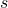for setting precision. We also have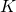as maximum integer value.

We want to decompose variable in the following form: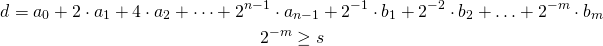We could take as many negative powers as possible. Now we add the following constraint: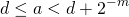It looks reasonable but it doesn’t work. Let’s take the following variables: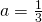,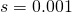. The result should be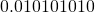in binary which gives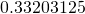. And now when we add lowest power, we get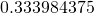. But when we now compare this by calculating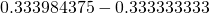we get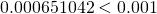. So we cannot determine those two numbers are different, we consider them equal!

But if we choose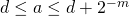then we have two representations of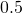. One is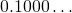, the other one is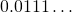. So with this approach we cannot approximate numbers reliably.